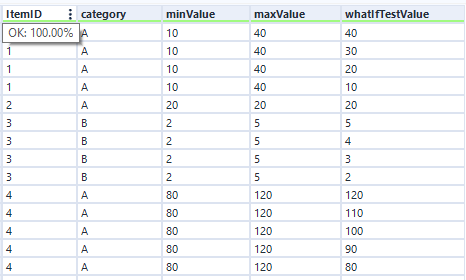## Generate Rows | Alteryx Tools Explained

by Harry Osborne

Generate Rows enables the user to create rows based on specified start and end points, or even to build a standalone table of values in a range. Furthermore, it has an added level of potential by utilising the inbuilt formula functions, both in the "Condition Expression" and the "Loop Expression".

Let's investigate this tool further, using the Alteryx examples as demos, and an Alteryx Weekly Challenge as an example use case.

### Explaining Generate Rows

Generate Rows works by creating new rows at the record level, allowing the creation of sequences, both in numerical and date form.

The set-up of the tool requires an understanding of several main parts. First, you can select to update an existing field or create a new field; the former will need a field to be selected to run, and the latter will ask for a data type and a field name to be input. Here, I am using the demo example, with a field named "RowCount".

Next, you will need to write in the "Initialisation Expression". This may appear at first to only take a string you yourself input (in this pictured example, I am going to use the number 1), but also takes fields you are using in your data if connected - these can be found under the ellipsis button to the right of the input box. This allows you to start from a dynamic point, as well as from a pre-existing value.

The complicated parts of the tool come in the final two steps. "Condition Expression" is where you specify the termination condition of the tool: for example, if you were to write "RowCount<=10" (as in the pictured example), the tool would stop running when that condition is no longer being met. Thus, when the value in field "RowCount" exceeds the number 10, the tool stops.

The final step (and arguably the most crucial one) is where you specify what the tool is actually going to do at each instance - i.e. during each "loop" (hence the name). As per my example, this configuration of Generate Rows is set up to add 1 to the existing value every loop. This is pretty much the most basic set up for this tool, but I will explain some more technical uses in more depth later on.

### #1: Generating a standalone table

This example is fairly simple: using the exact inputs I have shown in the images before, this setup will generate a table of rows from the values 1 through to 10, where the tool will finish running. This is useful as an append table, or as a lookup table for other data you may have in your Alteryx workflow. Furthermore, it requires zero pre-existing tables to use as a scaffold (whereas Record ID would).

### #2: Generating values in a range

Generate Rows comes into its own when filling out values between two specified points. This configuration of the tool pushes you to utilise pre-existing fields, but also requires you to have set up the data types correctly (e.g. using numerical fields if adding to an existing value). One such example was from the Alteryx Challenge for Week 1, where joining and filling between a range was required.

To create the values between Range1 and Range2 (i.e. for one Region/Sales Rep), I chose to create a new field named "Postal Area". From here, the "Initialisation Expression" I set to [Range 1] - i.e., the starting point for every one of the Ranges. I wanted this Generate Rows instance to end whenever it reached Range 2; thus, the "Condition Expression" I set to [Postal Area] <= [Range2], meaning that the tool would run unless the value in Postal Area was greater than the end point of the range.  Finally, the "Loop Expression" was relatively simple, merely adding 1 to the Postal Area value every increment.

As a result, the output gave me the Postal Area throughout the range 2000-2019 (and for all the other rows too). This entire setup can also be used with Date fields: for example, if wanting to increase the value per loop by 1 day, the formula in the "Loop Expression" would be DateTimeAdd([date],1,"days").

It is also worth noting that, given the formulaic configurations to these tools, they can work to variable degrees (i.e. run to different numbers of rows generated), so do not use inflexible, hard-coded values unless you want a consistent output.

### #3: Generating values using conditional formulae

Whilst every example so far has been A) unconditional and B) increasing, this final instance works the other way. Instead of having a linear expression inside the "Loop Expression", this next example works conditionally.

If [category]='A' Then [whatIfTestValue] - 10
ElseIf [category]='B' Then [whatIfTestValue] - 1
Else [minValue]-1 EndIf

Line by line, this formula works as follows:
LINE 1: If the Category is A, then the new value will be subtracted by 10
LINE 2: If the Category is B, the the new value will be subtracted by 1
LINE 3: If an unknown category appears, this logic forces the next value to be less than the minValue (the cutoff specified in the "Condition Expression"), so the loop stops.

Hence the output moves from this:

To this:Note the variable number of new rows and the different changes to their valuesHarry Osborne

Wed 14 Jul 2021

Wed 07 Jul 2021

Wed 07 Jul 2021

Wed 07 Jul 2021